##### Statistics For Dummies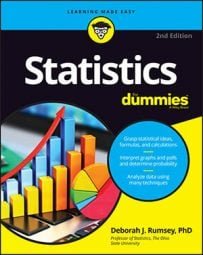In statistics, you can easily find probabilities for a sample mean if it has a normal distribution. Even if it doesn’t have a normal distribution, or the distribution is not known, you can find probabilities if the sample size, n, is large enough.

The normal distribution is a very friendly distribution that has a table for finding probabilities and anything else you need. For example, you can find probabilities for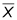by converting the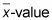to a z-value and finding probabilities using the Z-table (see below).

The general conversion formula from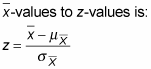Substituting the appropriate values of the mean and standard error ofthe conversion formula becomes: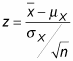Don’t forget to divide by the square root of n in the denominator of z. Always divide by the square root of n when the question refers to the average of the x-values.

For example, suppose X is the time it takes a randomly chosen clerical worker in an office to type and send a standard letter of recommendation. Suppose X has a normal distribution, and assume the mean is 10.5 minutes and the standard deviation 3 minutes. You take a random sample of 50 clerical workers and measure their times. What is the chance that their average time is less than 9.5 minutes?

This question translates to finding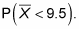also has an exact (not approximate) normal distribution. Converting to z, you get: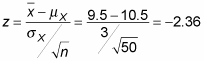So you want P(Z < –2.36).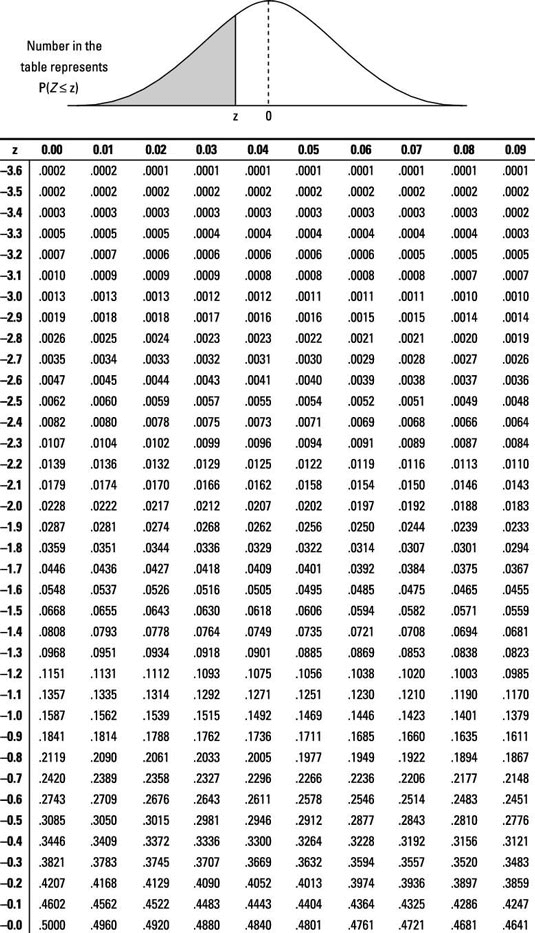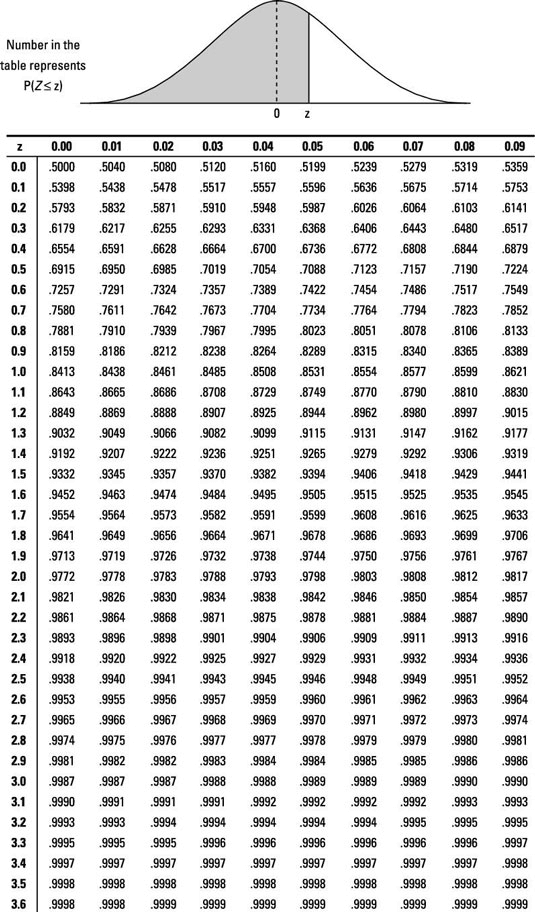Using the above Z-table, you find that P(Z < –2.36)=0.0091. So the probability that a random sample of 50 clerical workers average less than 9.5 minutes to complete this task is 0.91% (very small).

How do you find probabilities forif X is not normal, or unknown? As a result of the Central Limit Theorem (CLT), the distribution of X can be non-normal or even unknown and as long as n is large enough, you can still find approximate probabilities forusing the standard normal (Z-)distribution and the process described above. That is, convert to a z-value and find approximate probabilities using the Z-table.

When you use the CLT to find a probability for(that is, when the distribution of X is not normal or is unknown), be sure to say that your answer is an approximation. You also want to say the approximate answer should be close because you’ve got a large enough n to use the CLT. (If n is not large enough for the CLT, you can use the t-distribution in many cases.)